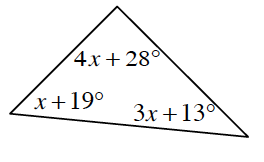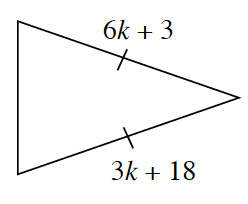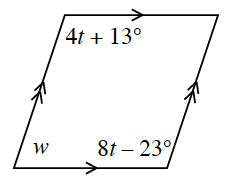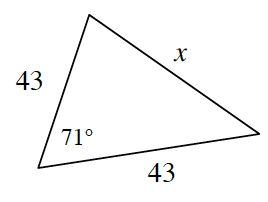### Home > CCG > Chapter 6 > Lesson 6.2.5 > Problem6-97

6-97.

For each shape below, use the geometric relationships to solve for the given variable(s). Show all work. Name the geometric relationships you used.

1.What is the sum of the angles within a triangle?

$x=15^\circ$, Triangle Angle Sum

1.What do the tick marks on the sides of the triangles mean?

$k=5$, Isosceles triangle

1.Extend the parallel sides.

Parallel lines have same side interior supplementary angles.

$\begin{array}{l} w+(4t+13)=180º\\ w+(8t−23)=180º \end{array}$

By the Equal Values Method: $w+(8t−23)=w+(4t+13)$

$\begin{array}{l} w+8(9)-23=180\\ w + 72 - 23 = 180\\ w + 49 = 180\\ w = 131º \end{array}$

$\begin{array}{r} 4t = 36\\ t = 9° \end{array}$

Parallel lines

1.Use the Law of Cosines to solve for the unknown side.